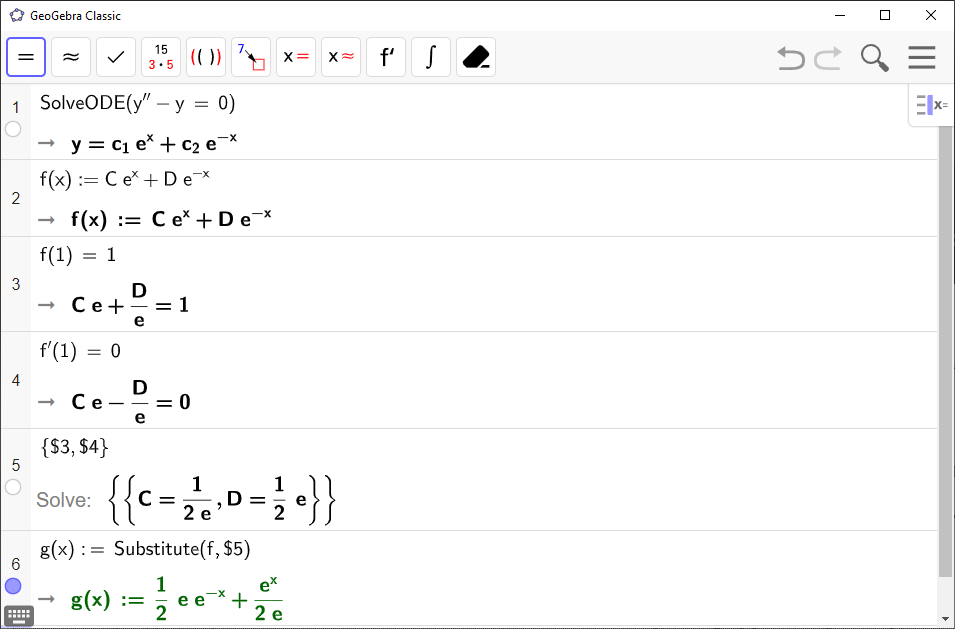# How to Write and Solve a Differential Equation in GeoGebra

You can use `GeoGebra` to find the general and particular solutions of a differential equation.

`GeoGebra` Instruction 1

Open `CAS` under`View` in`Menu`.

### General Solution

Type

SolveODE(<Equation>)

in `CAS`. For `<Equation>`, fill in the entire differential equation. Press `Enter`.

### Particular Solution

Type

SolveODE(<Equation>, <Point(s) on f>, <Point(s) on f’>)

in `CAS`. For `<Equation>`, fill in the entire differential equation. Type the initial condition $\left(a,f\left(a\right)\right)$ as `<Point(s) on f>`, and $\left(b,{f}^{\prime }\left(b\right)\right)$ as `<Point(s) on f’>`. Press `Enter`.